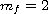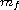Electron. J. Diff. Equ., Vol. 2014 (2014), No. 159, pp. 1-79.

### Geometric configurations of singularities for quadratic differential systems with total finite multiplicityJoan C. Artes, Jaume Llibre, Dana Schlomiuk, Nicolae Vulpe

Abstract:
In this work we consider the problem of classifying all configurations of singularities, both finite and infinite of quadratic differential systems, with respect to the geometric equivalence relation defined in . This relation is deeper than the topological equivalence relation which does not distinguish between a focus and a node or between a strong and a weak focus or between foci of different orders. Such distinctions are however important in the production of limit cycles close to the foci in perturbations of the systems. The notion of geometric equivalence relation of configurations of singularities allows to incorporates all these important geometric features which can be expressed in purely algebraic terms. This equivalence relation is also deeper than the qualitative equivalence relation introduced in . The geometric classification of all configurations of singularities, finite and infinite, of quadratic systems was initiated in  where the classification was done for systems with total multiplicityof finite singularities less than or equal to one. In this article we continue the work initiated in  and obtain the geometric classification of singularities, finite and infinite, for the subclass of quadratic differential systems possessing finite singularities of total multiplicity. We obtain 197 geometrically distinct configurations of singularities for this family. We also give here the global bifurcation diagram of configurations of singularities, both finite and infinite, with respect to the geometric equivalence relation, for this class of systems. The bifurcation set of this diagram is algebraic. The bifurcation diagram is done in the 12-dimensional space of parameters and it is expressed in terms of polynomial invariants. The results can therefore be applied for any family of quadratic systems in this class, given in any normal form. Determining the geometric configurations of singularities for any such family, becomes thus a simple task using computer algebra calculations.

Submitted November 25, 2013. Published July 18, 2014.
Math Subject Classifications: 58K45, 34C05, 34A34.
Key Words: Quadratic vector fields; infinite and finite singularities; affine invariant polynomials; Poincare compactification; configuration of singularities; geometric equivalence relation.

Show me the PDF file (2462 KB), TEX file, and other files for this article.Joan C. Artés Departament de Matemátiques Universitat Autónoma de Barcelona 08193 Bellaterra, Barcelona, Spain email: artes@mat.uab.cat Jaume Llibre Departament de Matemátiques Universitat Autónoma de Barcelona 08193 Bellaterra, Barcelona, Spain email: jllibre@mat.uab.cat Dana Schlomiuk Département de Mathématiques et de Statistiques Université de Montréal, Canada email: dasch@dms.umontreal.ca Nicolae Vulpe Institute of Mathematics and Computer Science Academy of Science of Moldova 5 Academiei str, Chisinau, MD-2028, Moldova email: nvulpe@gmail.com